# Three 43

Three brothers inherited a cash amount of 62,000 and they divided it among themselves in the ratio of 5:4:1. How much more is the largest share than the smallest share?

Result

x =  24800

#### Solution:

$p = 62000 \ \\ a = p \cdot \ \dfrac{ 5 }{ 5+4+1 } = 62000 \cdot \ \dfrac{ 5 }{ 5+4+1 } = 31000 \ \\ b = p \cdot \ \dfrac{ 4 }{ 5+4+1 } = 62000 \cdot \ \dfrac{ 4 }{ 5+4+1 } = 24800 \ \\ c = p \cdot \ \dfrac{ 1 }{ 5+4+1 } = 62000 \cdot \ \dfrac{ 1 }{ 5+4+1 } = 6200 \ \\ \ \\ x = a-c = 31000-6200 = 24800$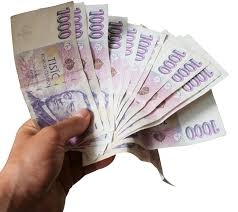Our examples were largely sent or created by pupils and students themselves. Therefore, we would be pleased if you could send us any errors you found, spelling mistakes, or rephasing the example. Thank you!

Leave us a comment of this math problem and its solution (i.e. if it is still somewhat unclear...):Be the first to comment!#### Following knowledge from mathematics are needed to solve this word math problem:

Need help calculate sum, simplify or multiply fractions? Try our fraction calculator.

## Next similar math problems:

1. Three workersThree workers got paid for work. First earn € 50, the second € 100 and third € 60. For further work should receive together € 735. How do you divide that amount in order to be distributed in the same proportion as the first job?
2. Babysitting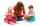The amount that Susan charges per hour for babysitting are directly proportional to the number of children she is watching. She charges $4.50 for 3 kids. How much would she charge to babysit 5 kids? 3. PeppersIn the box are yellow (a), green (b) and red (c) peppers. Their amount is in a ratio 2:4:1 . Most are yellow peppers and green the least. Calculate the number of peppers each type if the total number of peppers is 70. 4. Class 9.CProfessor collects money in the 9. C for a school trip. 2/3 of the collected amount was from girls and 1/4 from boys. The rest of 410 CZK went from the class fund. How much will the school trip cost in total? 5. Division of moneyCalculate how many euros have Matthew, Miriam, Lucy, Michael, Janka when together have 2,700 euros and the amounts are at a ratio of 1:5:6:7:8. 6. Moneys in triple ratioMilan, John and Lili have a total 344 euros. Their amounts are in the ratio 1:2:5. Determine how much each of them has? 7. DivideDivide the number 72 in the ratio 7: 2 and calculate the ratio of the numbers found in this order and write down as decimal. 8. Divide moneyDivide 1200 USD at a ratio of 1:2:3:4:5:6:9:10 9. Spending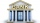Peter spends 1/5 of his earnings on his rent and he saves 2/7. What fraction of his earnings is left? 10. Land areaA land area of Asia and Africa are in a 3: 2 ratio, the European and African are is 1:3. What are the proportions of Asia, Africa, and Europe? 11. DonutsFind how many donuts each student will receive if you share 126 donuts in a ratio of 1:5:8 12. PamelaLara Pamela spends her salary of 3000 for food, clothing, recreation and savings, which are in the ratio of 48:20:15:37, respectively. How much does he spend for savings? 13. Line segmentThe 4 cm long line segment is enlarged in the ratio of 5/2. How many centimeters will measure the new line segment? 14. Hour salaryYou work for 4 hours on a Saturday and 8 hours on Sunday. You also receive a$50 bonus. You earn \$164. How much did you earn per hour?
15. The farmer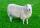The farmer had 140 sheep. For the next year, she decided to change the number of sheep in ratio 10: 7. How many sheep will he have then?
16. Image scale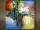The actual image dimensions are 60 cm x 80cm and has a reduced size 3 cm x 4 cm. At what scale the image was reduced?
17. AgesJohn, Teresa, Daniel and Paul have summary 56 years. Their ages are in a ratio of 1:2:5:6. Determine how many years have each of them.